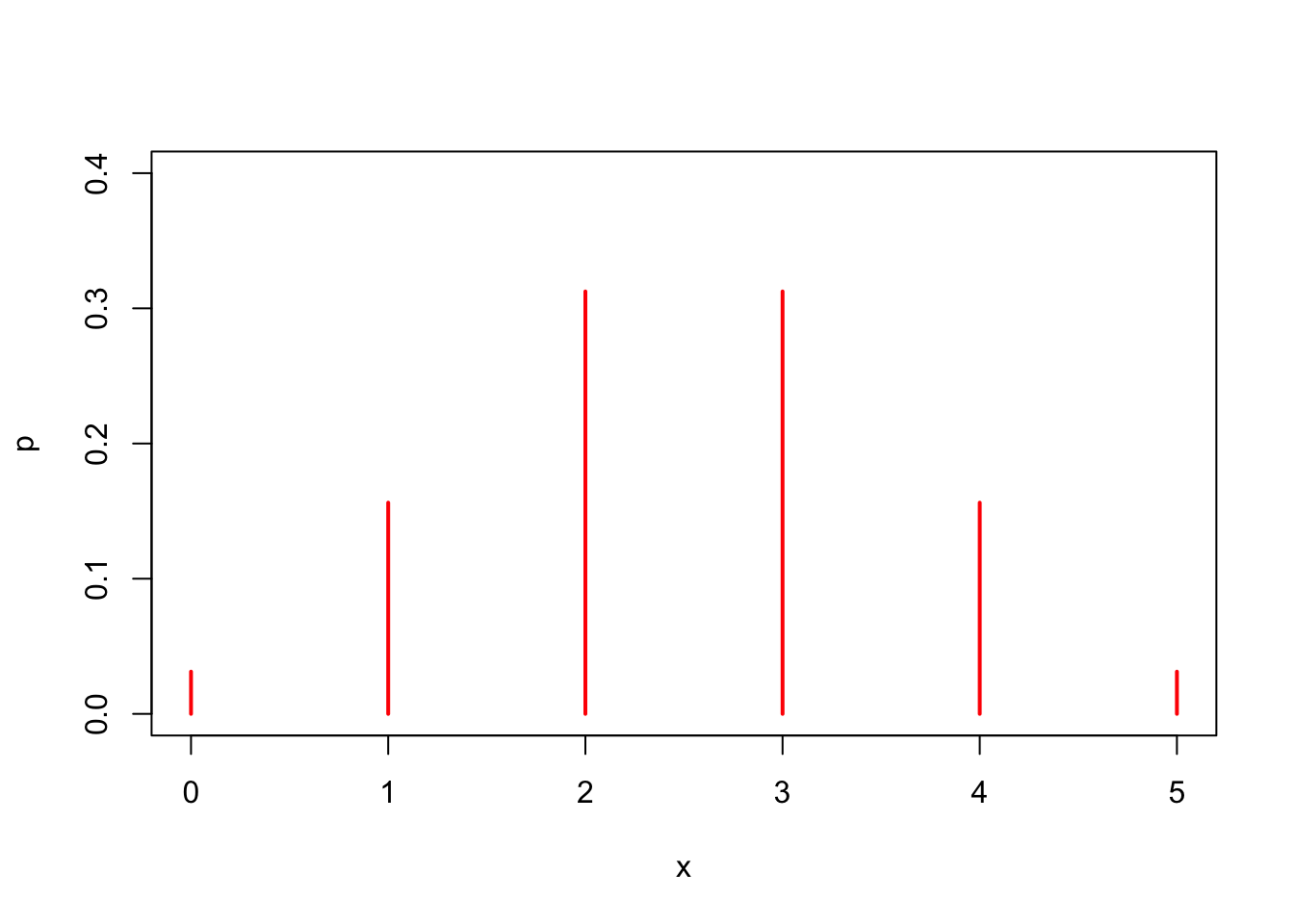In this activity we will learn how to find the mean, variance, and standard deviation of a binomial distribution. We will use the example of tossing a fair coin $$n=5$$ times, then calculating the probability of tossing $$X=0$$, 1, 2, 3, 4, or 5 heads. First, set the number of tosses and the probability of tossing a head.

n=5
prob=0.5

Next, the possibilities are tossing $$X=0$$, 1, 2, 3, 4, or 5 head. Set $$X$$ as follows.

x=0:5
x
##  0 1 2 3 4 5

Next, we use R’s dbinom command to compute each probability of tossing $$X=0$$, 1, 2, 3, 4, or 5 heads.

p=dbinom(x,n,prob)
p
##  0.03125 0.15625 0.31250 0.31250 0.15625 0.03125

### Creating a Stick Graph

Now we will create a stick graph for our data.

plot(x,p,type='h',
ylim=c(0,0.4),
lwd=2,
col="red")Based on the image, the balance point (mean) appears to be around 2.5.

### Using a Table to Calculate $$\mu$$, $$\sigma^2$$, and $$\sigma$$.

We will use R’s cbind command to store the columns $$x$$, $$p$$, $$xp$$, and $$x^2p$$.

data=cbind(x,p,x*p,x^2*p)
data
##      x       p
## [1,] 0 0.03125 0.00000 0.00000
## [2,] 1 0.15625 0.15625 0.15625
## [3,] 2 0.31250 0.62500 1.25000
## [4,] 3 0.31250 0.93750 2.81250
## [5,] 4 0.15625 0.62500 2.50000
## [6,] 5 0.03125 0.15625 0.78125

We would like to add some row names and column names.

rownames(data)=0:5
colnames(data)=c("x","p","xp","x^2p")
data
##   x       p      xp    x^2p
## 0 0 0.03125 0.00000 0.00000
## 1 1 0.15625 0.15625 0.15625
## 2 2 0.31250 0.62500 1.25000
## 3 3 0.31250 0.93750 2.81250
## 4 4 0.15625 0.62500 2.50000
## 5 5 0.03125 0.15625 0.78125

Now we would like to sum the columns.

addmargins(data,1)
##      x       p      xp    x^2p
## 0    0 0.03125 0.00000 0.00000
## 1    1 0.15625 0.15625 0.15625
## 2    2 0.31250 0.62500 1.25000
## 3    3 0.31250 0.93750 2.81250
## 4    4 0.15625 0.62500 2.50000
## 5    5 0.03125 0.15625 0.78125
## Sum 15 1.00000 2.50000 7.50000

### Computing Mean, Variance, and Standard Deviation

Now we can compute the mean, using the formula $$\mu=\sum xp$$.

mu=sum(x*p)
mu
##  2.5

Aha! That agrees with the guess we made based on our stick graph. Now, let’s compute the variance, using the formula $$\sigma^2=\sum x^2p-\mu^2$$.

sigma.sq=sum(x^2*p)-mu^2
sigma.sq
##  1.25

Hence, the variance is $$\sigma^2=1.25$$. To find the standard deviation, we take the square root of the variance.

sigma=sqrt(sigma.sq)
sigma
##  1.118034

Hence, the standard deviation is $$\sigma=1.118034$$.

### Is There a Quicker Way?

There is a much quicker way to calculate the mean, variance, and standard deviation of a binomial distribution using the formulas \begin{align*} \mu&=np\\ \sigma^2&=npq\\ \sigma&=\sqrt{npq}, \end{align*} where $$n$$ represents the number of trials (e.g., the number of coin tosses), $$p$$ represents the probability of success (e.g., the probability of tossing a head), and $$q$$ represents the probability of failure (e.g., the probability of not tossing a head (i.e., a tail)).

Above, we use prob as a variable for the probability of success. Let change that to $$p$$ for these calculations. So, we toss a coin $$n=5$$ times and the probability of getting a head is $$p=0.5$$. Note that $$q$$, the probability of failure, is $$a=1-p$$.

n=5
p=0.5
q=1-p
c(n,p,q)
##  5.0 0.5 0.5

Let’s use the first formula to calculate the mean and see if it agrees with our result calculated above.

mu=n*p
mu
##  2.5

Yay! We got 2.5 again. Now, let’s get the variance using the second formula.

sigma.sq=n*p*q
sigma.sq
##  1.25

Yay! That is exactly the same number we got above. Finally, calculate the standard deviation using the third formula.

sigma=sqrt(n*p*q)
sigma
##  1.118034

Yay! Again, the same answer as calculated above.

### Easier Formulas

The formulas \begin{align*} \mu&=np\\ \sigma^2&=npq\\ \sigma&=\sqrt{npq} \end{align*} are a much simpler way to calculate the mean, variance, and standard deviation of a binomial distribution.